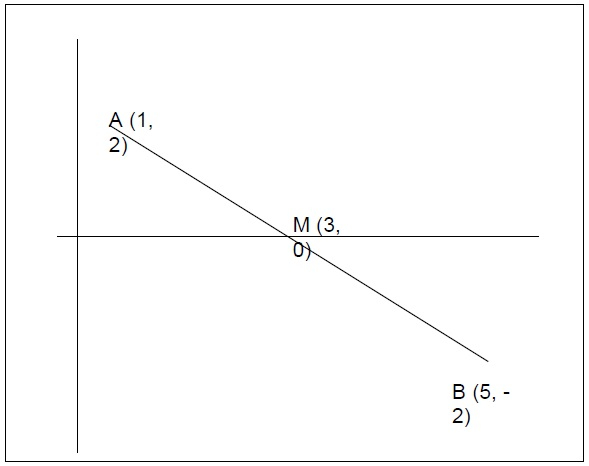# Find the other end point of a line with given one end and mid using C++

In this problem, we are given the coordinates of two points of a line starting point A(xA, yA) and midpoint M(xM, yM) .Our task is to find the other end point of a line with given one end and mid.

Let’s take an example to understand the problem,

Input

A = [1, 2], M = [3, 0]

Output

[5, -2]

## Explanation

The line is −## Solution Approach

To solve the problem, we will be using the concepts of geometry we have learned in mathematics. If you remember there is a midpoint formula for every line which is,

mid(x) = (x1 + x2) / 2
mid(y) = (y1 + y2) / 2

But we are given the value of the midpoint in the problem and need the value for x2 and y2. So, we will change the formula accordingly.

x2 = 2*mid(x) - x1
y2 = 2*mid(y) - y1

Using the above formula, we can find the value of the other endpoint using the midpoint and one point of the line.

## Example

Program to illustrate the working of our solution

#include <iostream>
using namespace std;
void findMissingPointLine(float x1, float y1, float xm, float ym){
float x2 = (2 * xm) - x1;
float y2 = (2 * ym) - y1;
cout<<"B(x, y) = "<<"( "<<x2<<", "<<y2<<" )";
}
int main()
{
float x1 = -4, y1 = -1, xm = 3, ym = 5;
cout<<"The other end point of the line is \n";
findMissingPointLine(x1, y1, xm, ym);
return 0;
}

## Output

The other end point of the line is
B(x, y) = ( 10, 11 )